Next: Worked Example 3: Motional Up: Magnetic Induction Previous: Example 9.1: Faraday's law

## Worked Example 2: Lenz's law

Question: A long solenoid with an air core has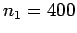turns per meter and a cross-sectional area of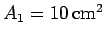. The current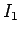flowing around the solenoid increases from 0 to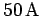in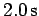. A plane loop of wire consisting of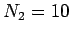turns, which is of cross-sectional area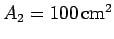and resistance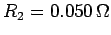, is placed around the solenoid close to its centre. The loop is orientated such that it lies in the plane perpendicular to the axis of the solenoid. What is the magnitude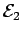of the emf induced in the coil? What current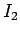does does this emf drive around the coil? Does this current circulate in the same direction as the current flowing in the solenoid, or in the opposite direction?

Answer: We must, first of all, calculate the magnetic flux linking the coil. The magnetic field is confined to the region inside the solenoid (the field generated outside a long solenoid is essentially negligible). The magnetic field runs along the axis of the solenoid, so it is directed perpendicular to the plane of the coil. Thus, the magnetic flux linking a single turn of the coil is the product of the area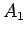of the magnetic-field-containing region and the strengthof the perpendicular field. Note that, in this case, the magnetic flux does not depend on the area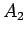of the coil, as long as the magnetic-field-containing region lies completely within the coil. The magnetic flux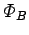linking the whole coil is the flux linking a single turn times the number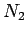of turns in the coil. Thus,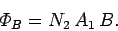Now, the magnitude of the magnetic field generated by the solenoid is given by (see Sect. 8.8)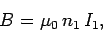so the magnetic flux linking the coil can be written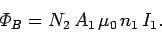This magnetic flux increases because the currentflowing in the solenoid increases. Thus, the time rate of change of the magnetic flux is given by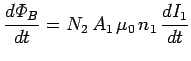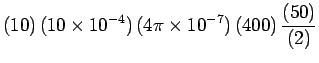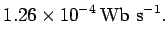By Faraday's induction law, the emf generated around the coil is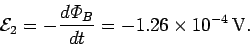Ohm's law gives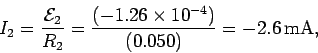as the current induced in the coil.

According to Lenz's law, the current induced in the coil is such as to oppose the increase in the magnetic flux linking the coil. Thus, the current in the coil must circulate in the opposite direction to the current in the solenoid, so that the magnetic field generated by the the former current, in the middle of the coil, is oppositely directed to that generated by the latter current. The fact that the currentin the above formula is negative is indicative of the fact that this current runs in the opposite direction to the current flowing around the solenoid.Next: Worked Example 3: Motional Up: Magnetic Induction Previous: Example 9.1: Faraday's law
Richard Fitzpatrick 2007-07-14